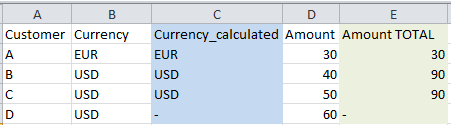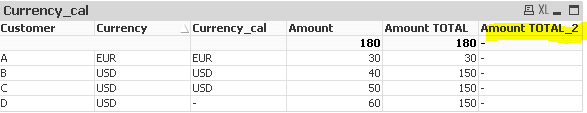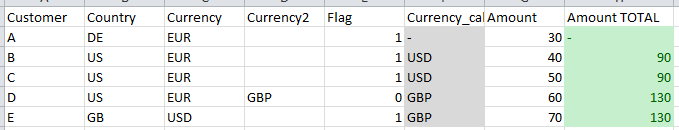# QlikView App Development

Discussion Board for collaboration related to QlikView App Development.

New Contributor III

## SUM TOTAL by expression values

Hello,

is it possible to sum up values by the output of another expression using the TOTAL qualifier? Example:Column C is an calculated expression based on column B. I would like to aggregate the amount (column D) by the output of column C. The desired output is displayed in column E. In Qlikview, I am trying to achieve this result using the function TOTAL:However, I receive the following error: "Total specifier must be a valid field name" .Is there another way to solve this problem? Please find attached the qvw containing the example.

Please also note that this is an simplified example and in reality the currency calculation is very complex containing several IF-statements so it is not possible to use the whole calculation within the total function (total<original expression>).

Many thanks,

Paul

Tags (2)
1 Solution

Accepted SolutionsLuminary

## Re: SUM TOTAL by expression values

Hi Paul,

First, I would assume that TOTAL qualified (like set analysis) is not evaluated on row level, but on chart level, so I guess you're not able to make it dynamic using conditions. Second, it expects field name as a input. Your variable returns currency code (which is not a field name). I remember trying to use table column in TOTAL without success and I rewrote the expression using creating separate Sum(TOTAL) for each scenario, summing them up by RangeSum() (I might have used Aggr() as well, don't rememnber, it was some time ago).

So, my recomendation is to calculate an extra field in script, which would contain proper currency for this aggregation, and you don't need to do this kind of gymnastics.

Hope this helps.

Juraj

6 RepliesMVP

## Re: SUM TOTAL by expression values

Try this

If(Match(Customer,'A','B','C'), Sum(TOTAL <Currency> {<Customer = {'A', 'B', 'C'}>}Amount))MVP

## Re: SUM TOTAL by expression values

Try this?

If(Match(Customer,'A','B','C'), Sum(TOTAL <Currency> {<Customer = {'A', 'B', 'C'}>}Amount))

Before develop something, think If placed (The Right information | To the right people | At the Right time | In the Right place | With the Right context)
New Contributor III

## Re: SUM TOTAL by expression values

Thank you!

My currency calculation is more complex, so I have updated the example. Outcome should be as follows (green column):Luminary

## Re: SUM TOTAL by expression values

Hi Paul,

First, I would assume that TOTAL qualified (like set analysis) is not evaluated on row level, but on chart level, so I guess you're not able to make it dynamic using conditions. Second, it expects field name as a input. Your variable returns currency code (which is not a field name). I remember trying to use table column in TOTAL without success and I rewrote the expression using creating separate Sum(TOTAL) for each scenario, summing them up by RangeSum() (I might have used Aggr() as well, don't rememnber, it was some time ago).

So, my recomendation is to calculate an extra field in script, which would contain proper currency for this aggregation, and you don't need to do this kind of gymnastics.

Hope this helps.

JurajMVP

## Re: SUM TOTAL by expression values

I agree with juraj.misina‌, if this is something you can do in the script (creating currency_cal in the script), then you will save yourself from a very complicated expression (not even sure this is possible to do yet).

Highlighted
New Contributor III

## Re: SUM TOTAL by expression values

Hi Juray,

thanks for your input - I have been afraid it's to complicated for an expression. However, it's a little odd that it's not possible to use calculated values for aggregation, since the result is available on row level.

Cheers,

Paul